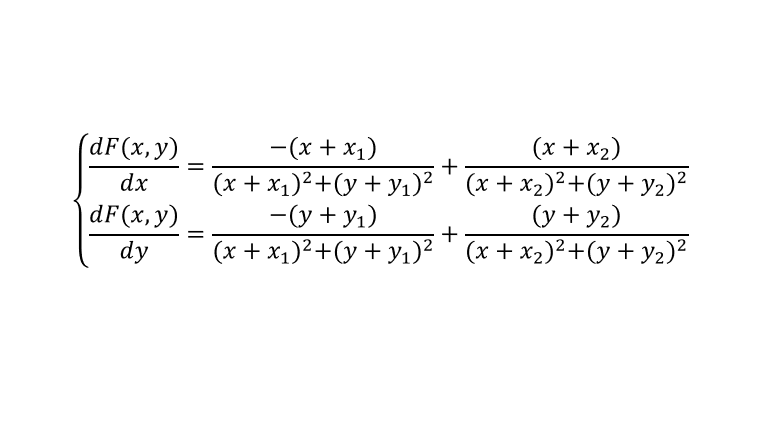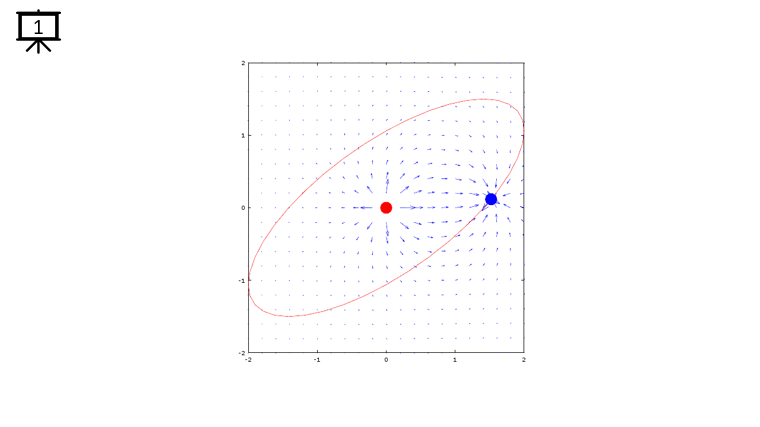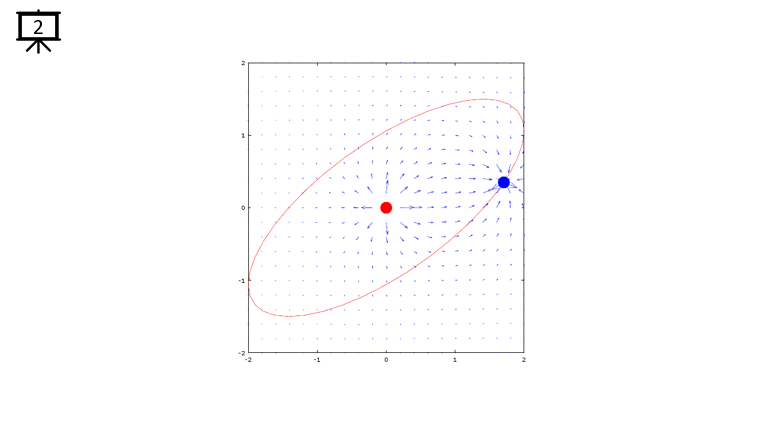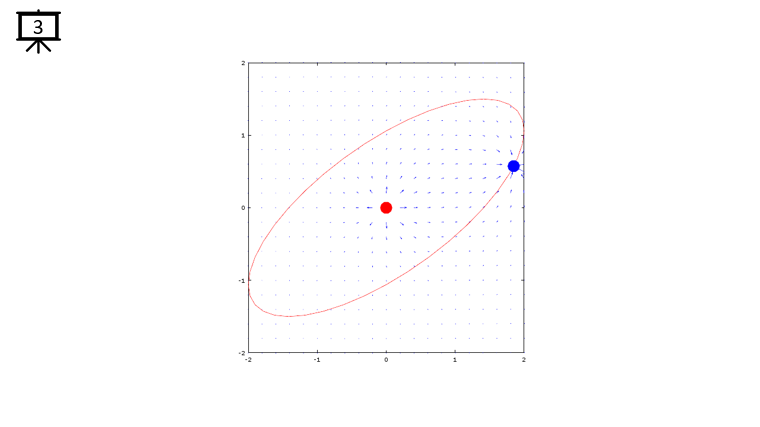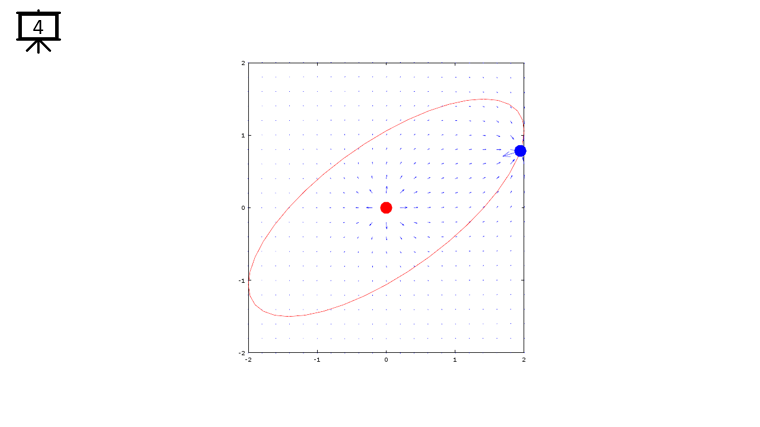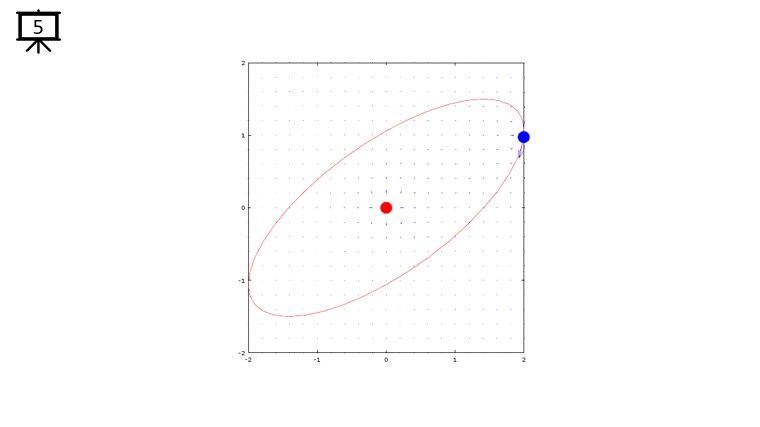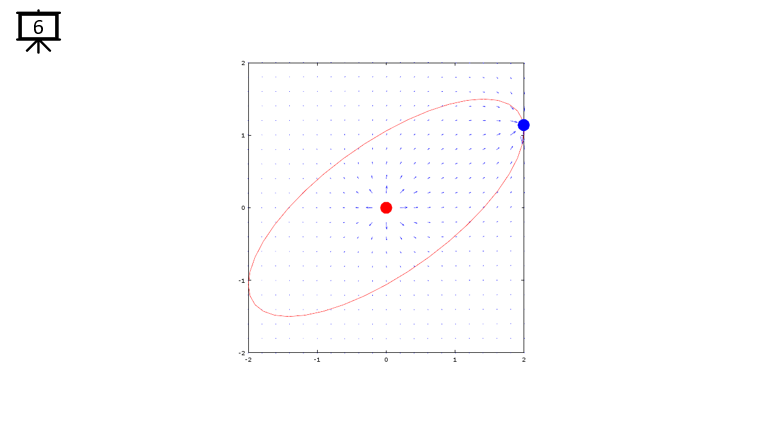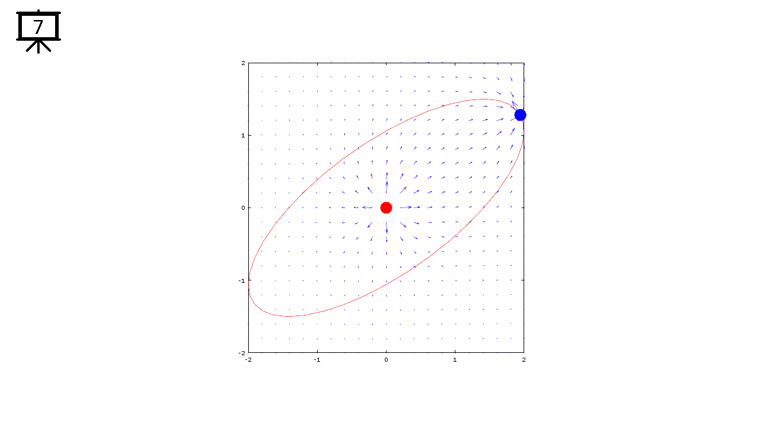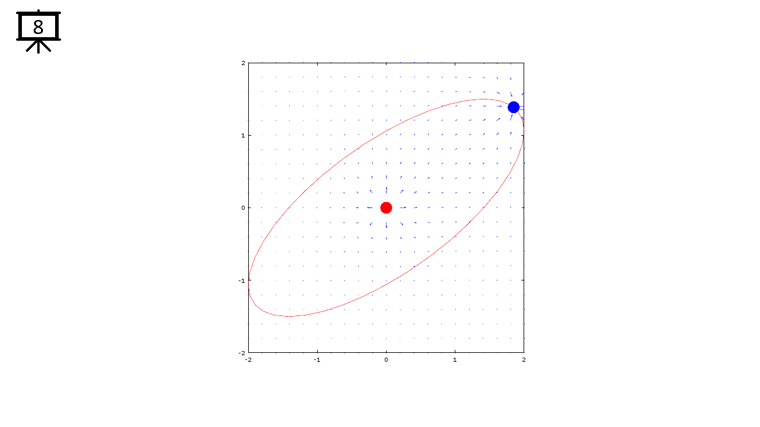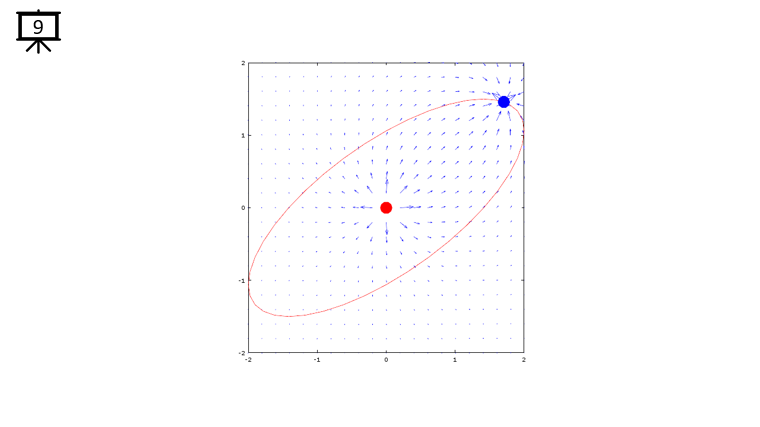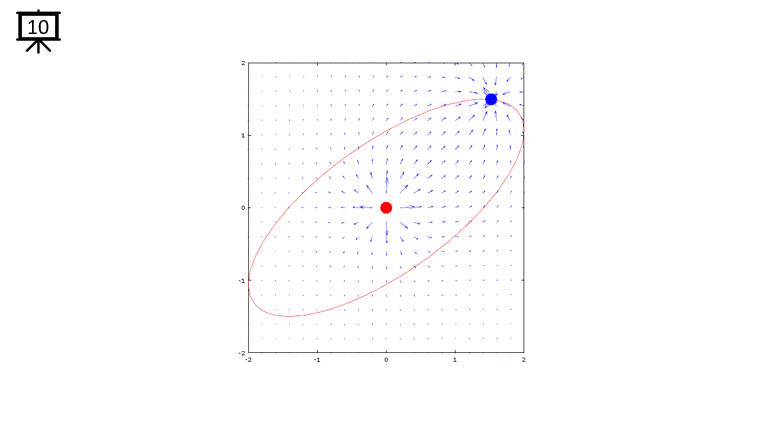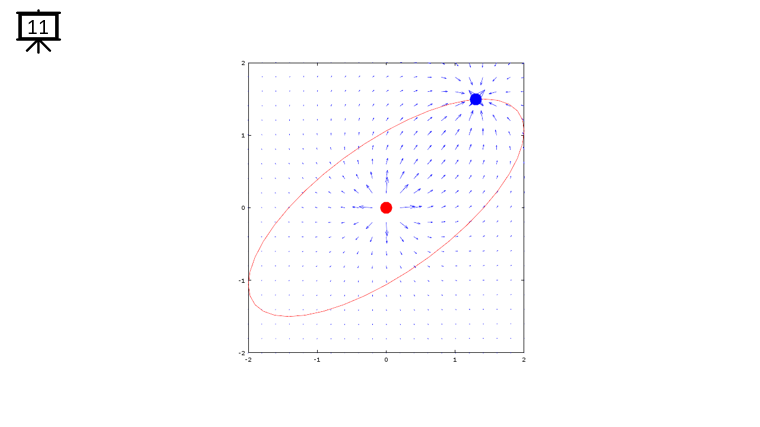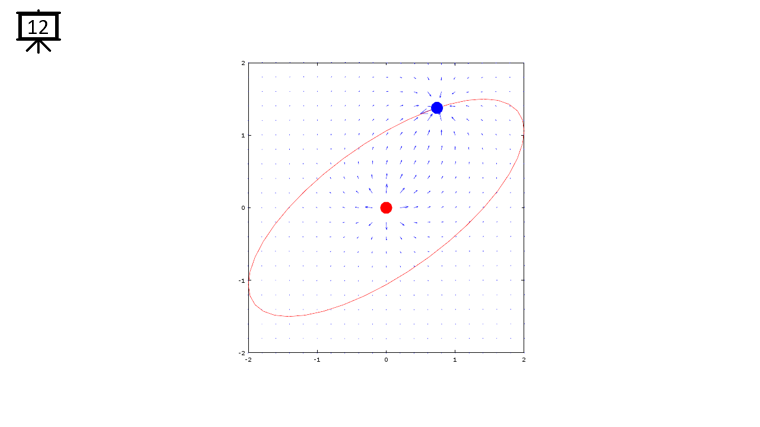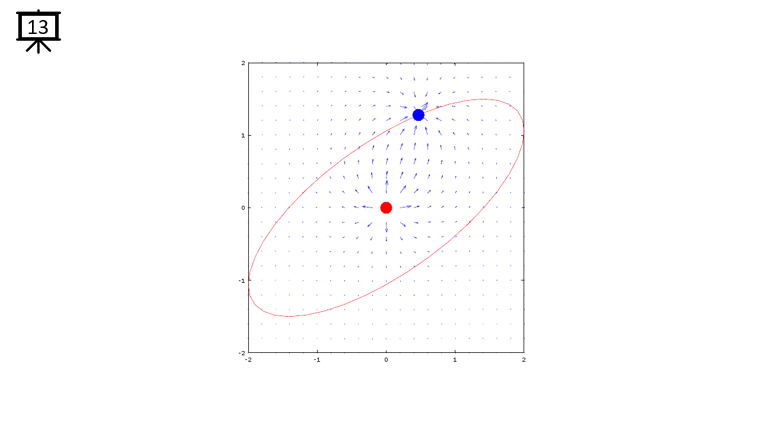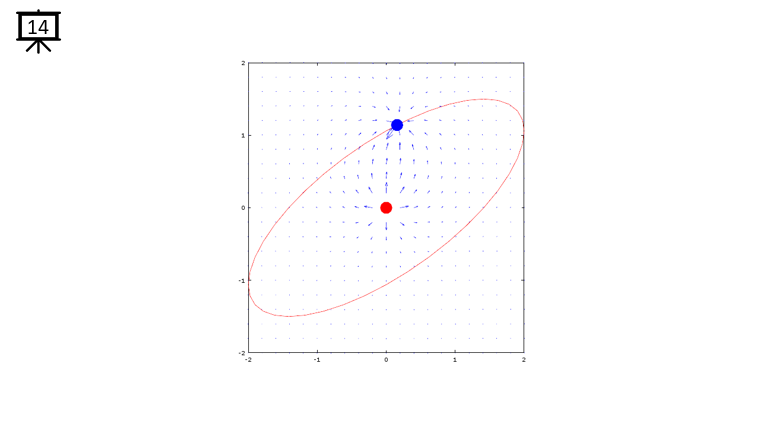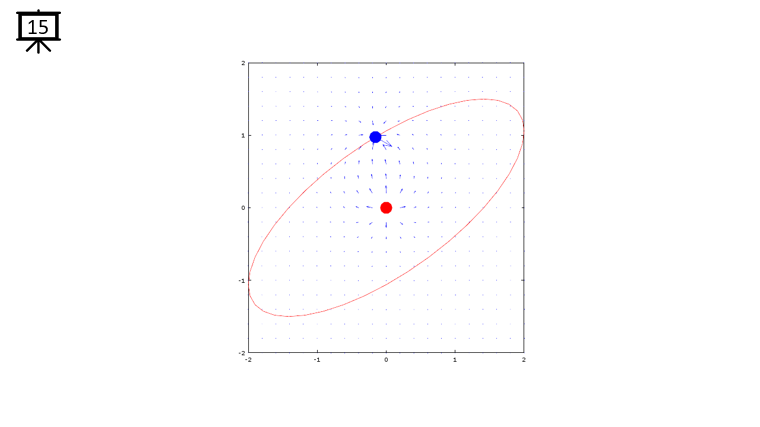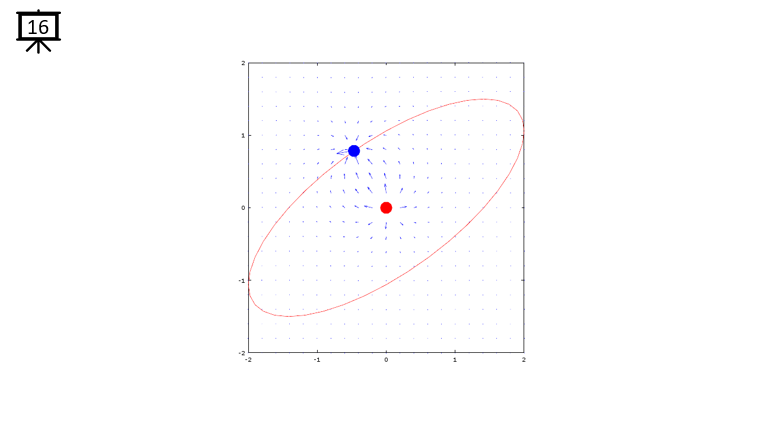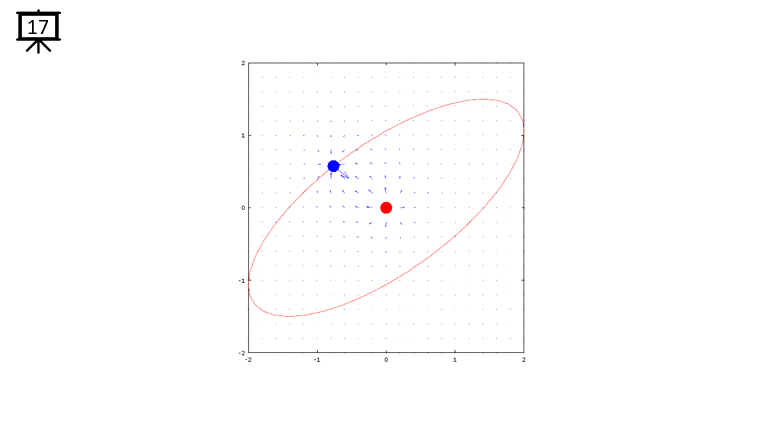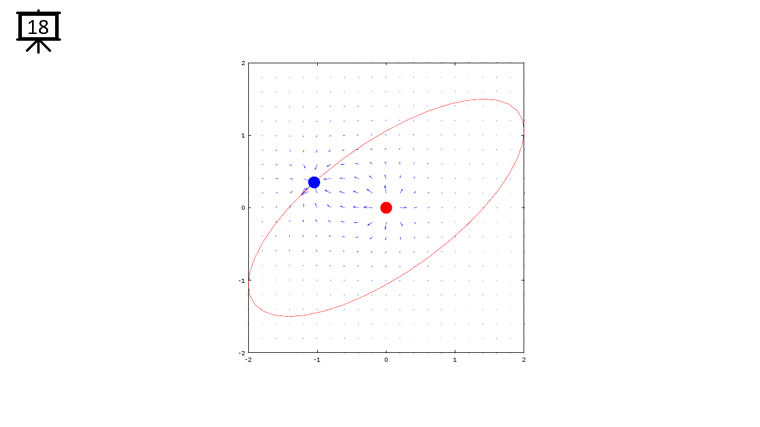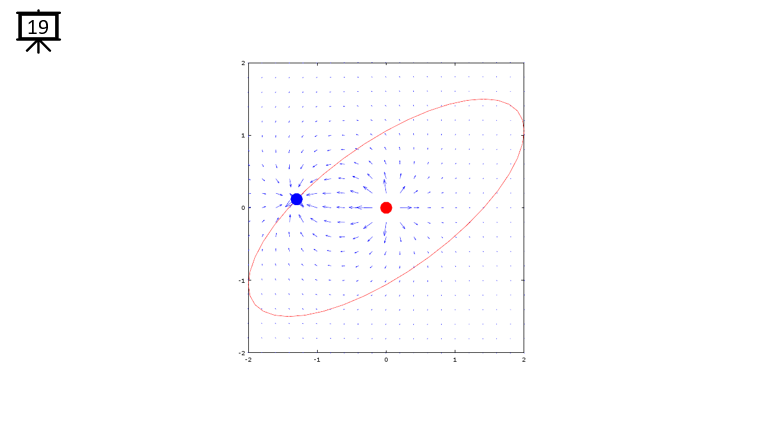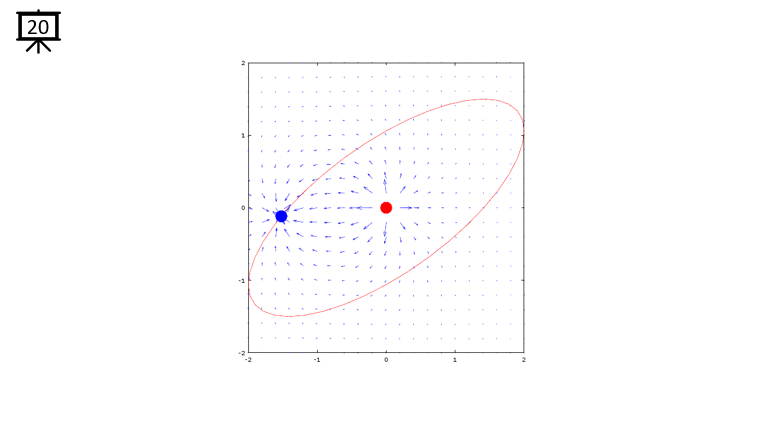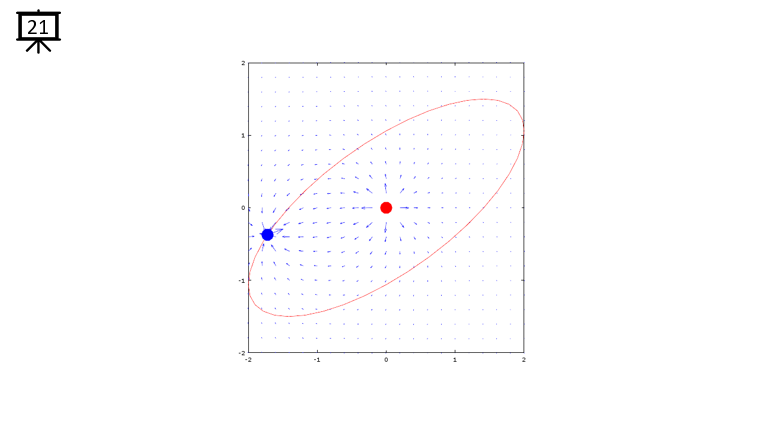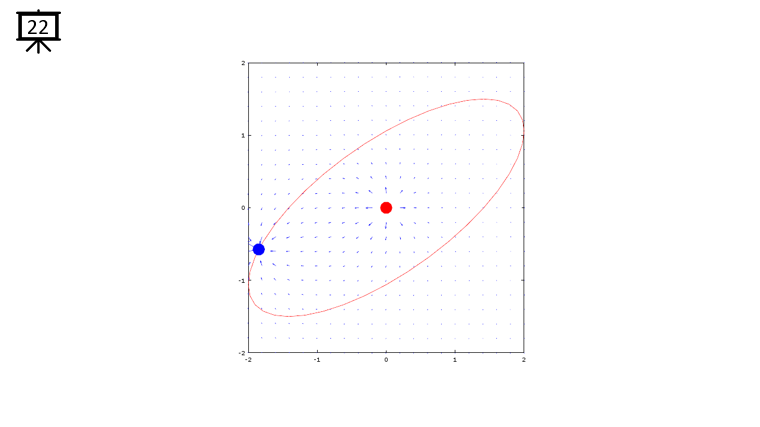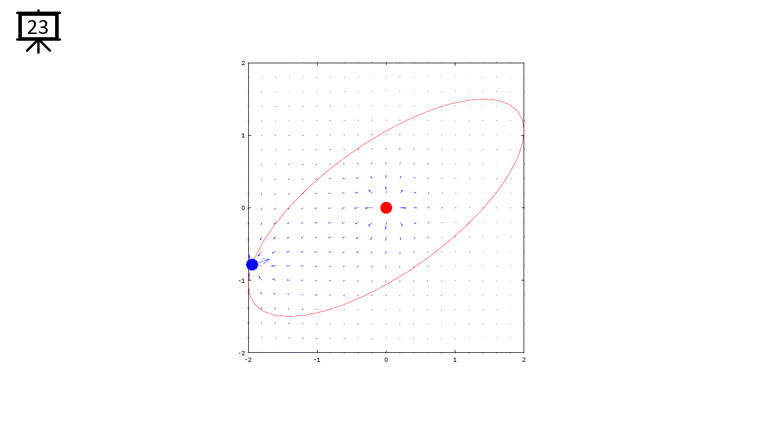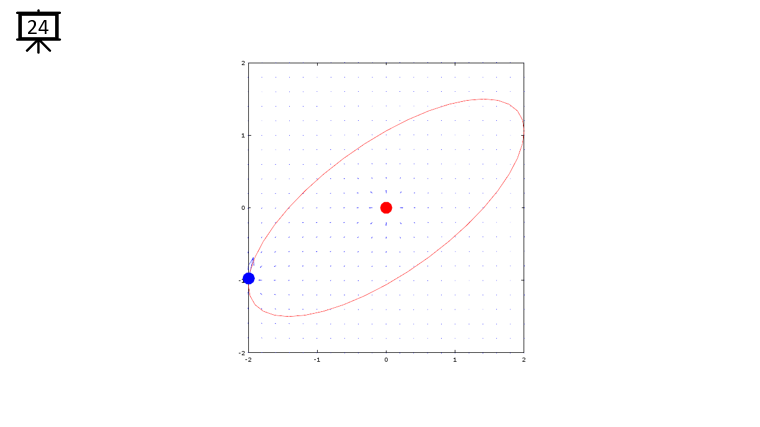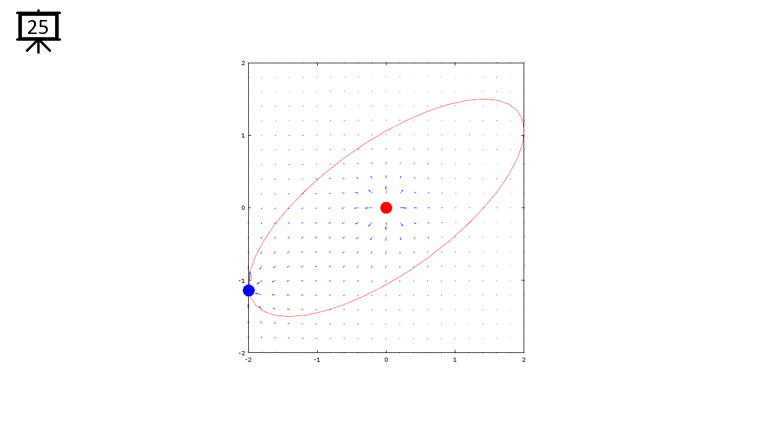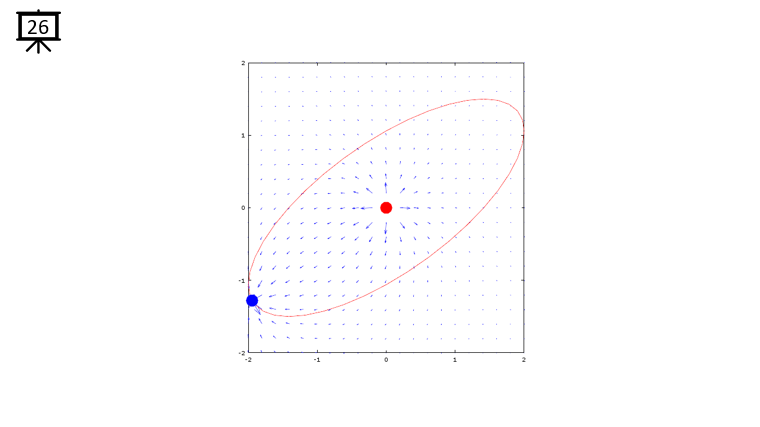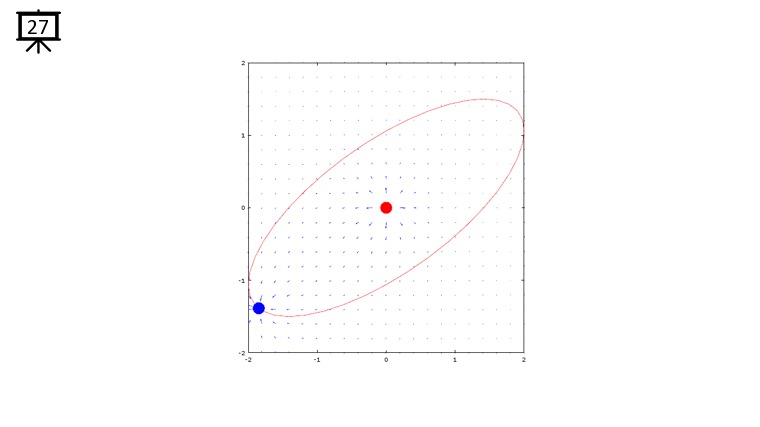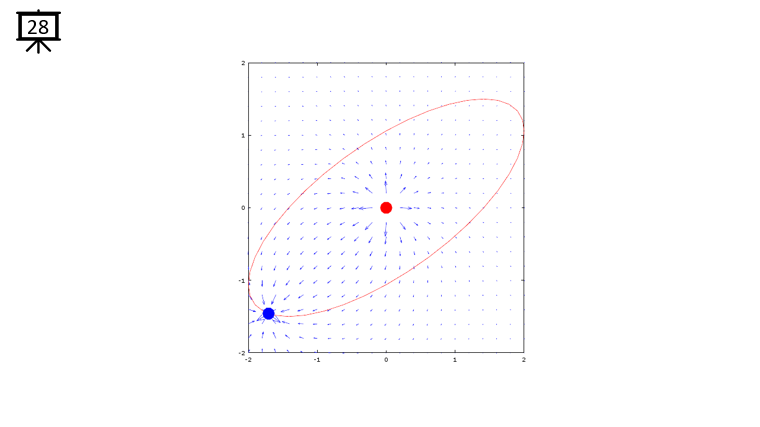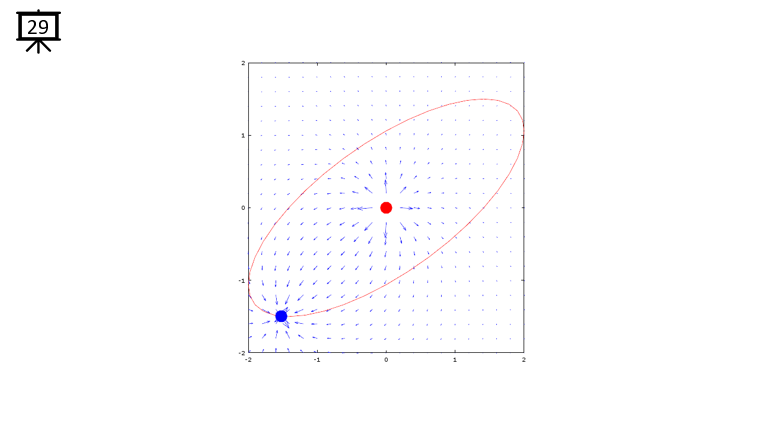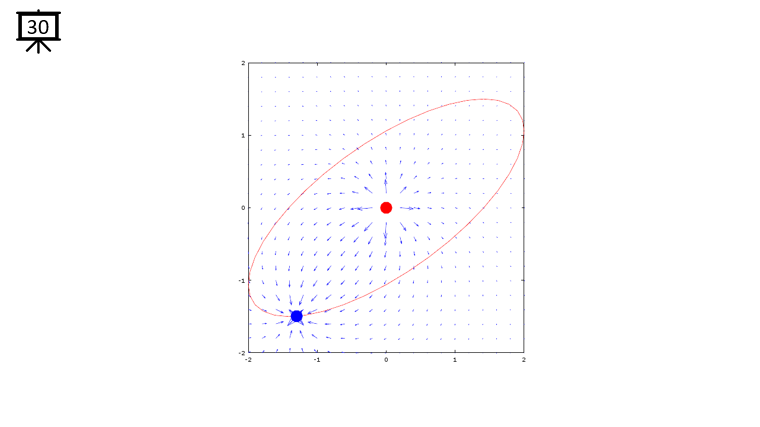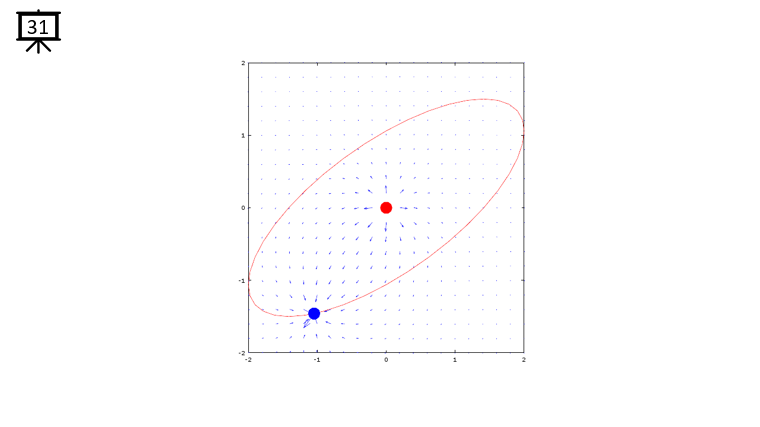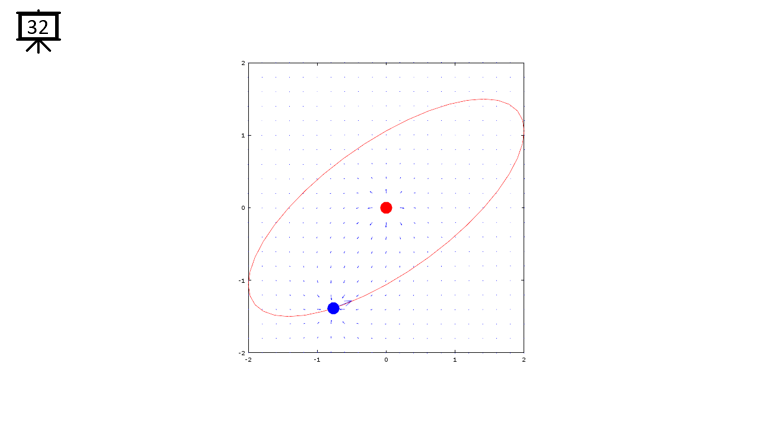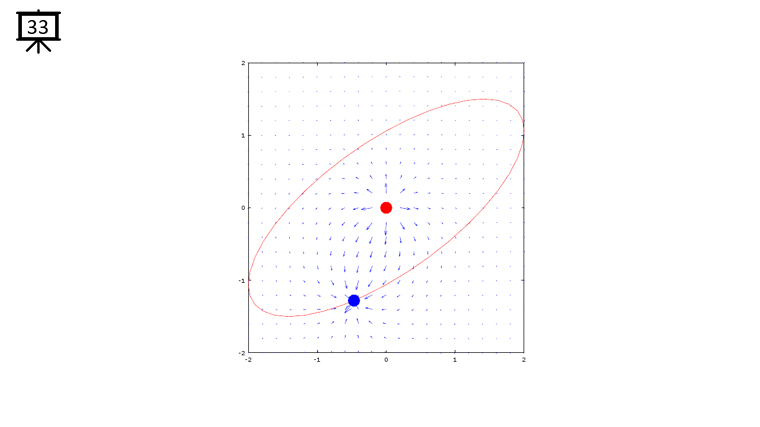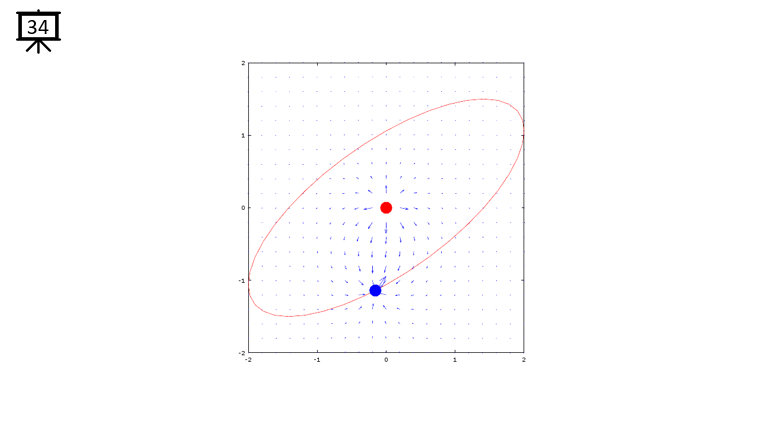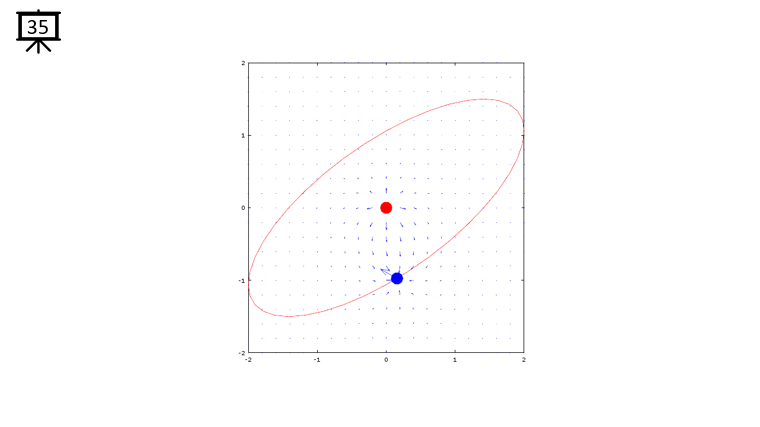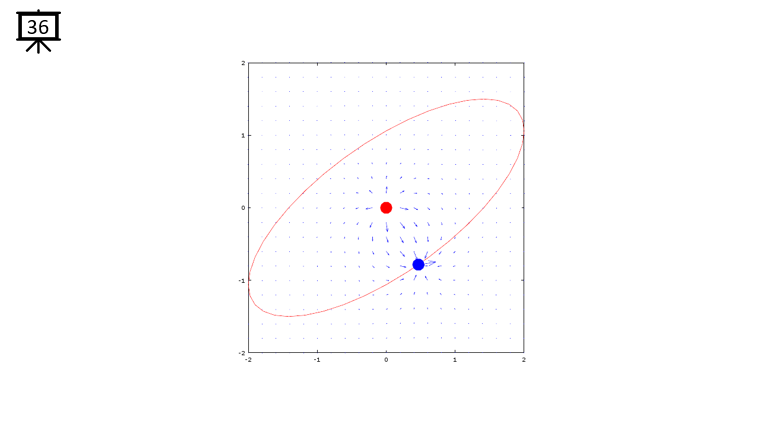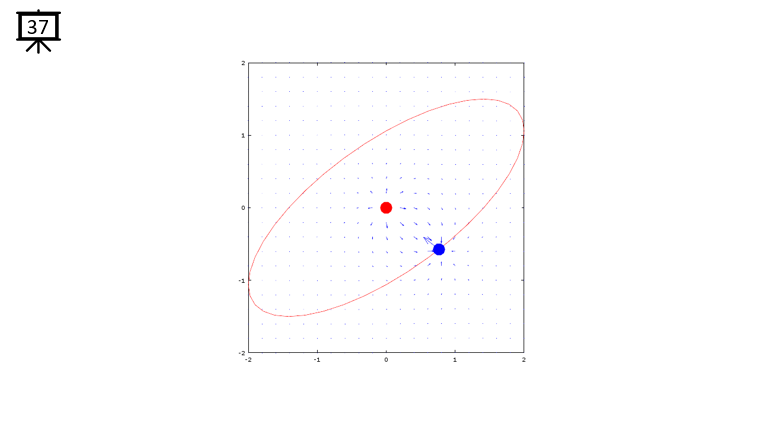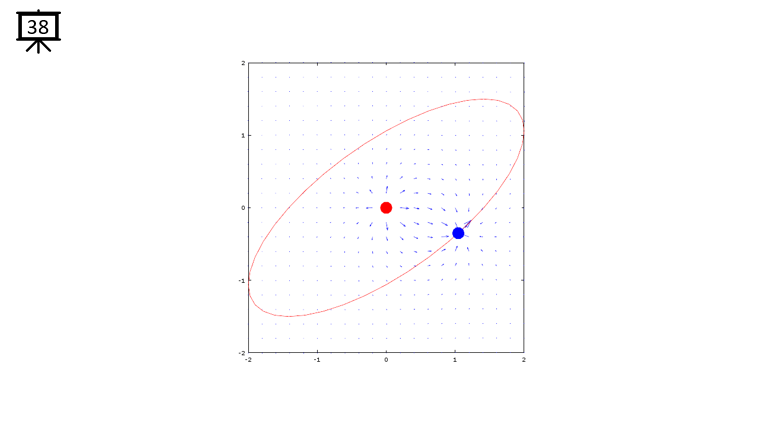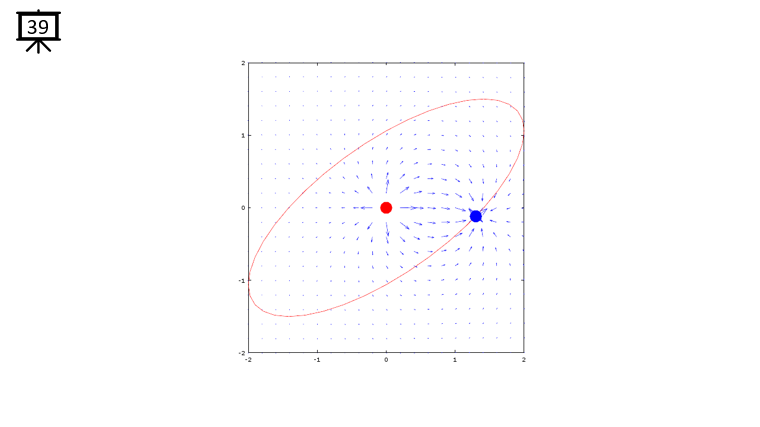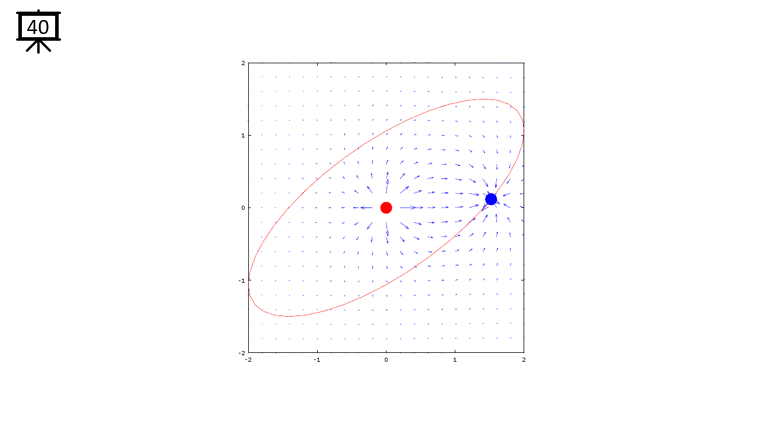Example of Vector Field - Two Charges

 Followings are the code that I wrote in Octave to creates all the plots shown in this page. You may copy these code and play with these codes. Change variables and try yourself until you get your own intuitive understanding.   < Code 1 >   v = -2:0.2:2;  % you can change the range to best fit the plot th = 0:pi/20:2*pi; xth = 2*cos(th-pi/4); yth = 1.5*sin(th); t0 = (0.5+40.0) * pi/20; a = -1; b = 1; c = -1; d = 1; x1 = -2*cos(t0-pi/4); x2 = 0; y1 = -1.5*sin(t0); y2 = 0;   [x,y] = meshgrid(v);   dx= a .* (x+x1) ./ ((x+x1) .^2 + (y+y1) .^2) + b .* (x+x2) ./ ((x+x2) .^2 + (y+y2) .^2); dy= c .* (y+y1) ./ ((x+x1) .^2 + (y+y1) .^2) + d .* (y+y2) ./ ((x+x2) .^2 + (y+y2) .^2);   hFig = figure(1); hold on; quiver(x,y,dx,dy,1.2); plot(-x2,-y2,'ro','MarkerFaceColor',[1 0 0],'MarkerSize',20); plot(-x1,-y1,'bo','MarkerFaceColor',[0 0 1],'MarkerSize',20); plot(xth,yth,'r-'); axis([-2 2 -2 2]); hold off; set(hFig,'Position',[300 100 600 600]);